Next: Low Energy Scattering Up: Scattering Theory Previous: Determination of Phase-Shifts

# Hard Sphere Scattering

Let us test out this scheme using a particularly simple example. Consider scattering by a hard sphere, for which the potential is infinite for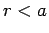, and zero for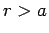. It follows thatis zero in the region, which implies that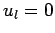for all. Thus,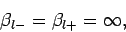(1319)

for all. Equation (1312) thus gives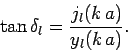(1320)

Consider thepartial wave, which is usually referred to as the-wave. Equation (1320) yields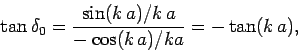(1321)

where use has been made of Eqs. (1289) and (1290). It follows that(1322)

The-wave radial wave function is [see Eq. (1310)]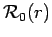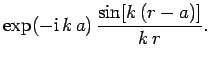(1323)

The corresponding radial wavefunction for the incident wave takes the form [see Eq. (1298)]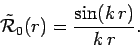(1324)

Thus, the actualradial wavefunction is similar to the incidentwavefunction, except that it is phase-shifted by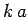.

Let us examine the low and high energy asymptotic limits of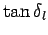. Low energy implies that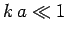. In this regime, the spherical Bessel functions reduce to: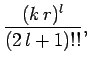(1325)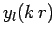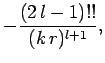(1326)

where. It follows that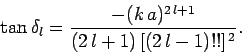(1327)

It is clear that we can neglect, with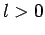, with respect to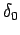. In other words, at low energy only-wave scattering (i.e., spherically symmetric scattering) is important. It follows from Eqs. (1266), (1306), and (1322) that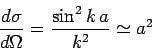(1328)

for. Note that the total cross-section(1329)

is four times the geometric cross-section(i.e., the cross-section for classical particles bouncing off a hard sphere of radius). However, low energy scattering implies relatively long wavelengths, so we would not expect to obtain the classical result in this limit.

Consider the high energy limit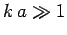. At high energies, all partial waves up to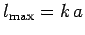contribute significantly to the scattering cross-section. It follows from Eq. (1308) that(1330)

With so manyvalues contributing, it is legitimate to replaceby its average value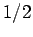. Thus,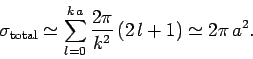(1331)

This is twice the classical result, which is somewhat surprizing, since we might expect to obtain the classical result in the short wavelength limit. For hard sphere scattering, incident waves with impact parameters less thanmust be deflected. However, in order to produce a shadow'' behind the sphere, there must also be some scattering in the forward direction in order to produce destructive interference with the incident plane-wave. In fact, the interference is not completely destructive, and the shadow has a bright spot (the so-called Poisson spot'') in the forward direction. The effective cross-section associated with this bright spot iswhich, when combined with the cross-section for classical reflection,, gives the actual cross-section of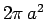.Next: Low Energy Scattering Up: Scattering Theory Previous: Determination of Phase-Shifts
Richard Fitzpatrick 2010-07-20